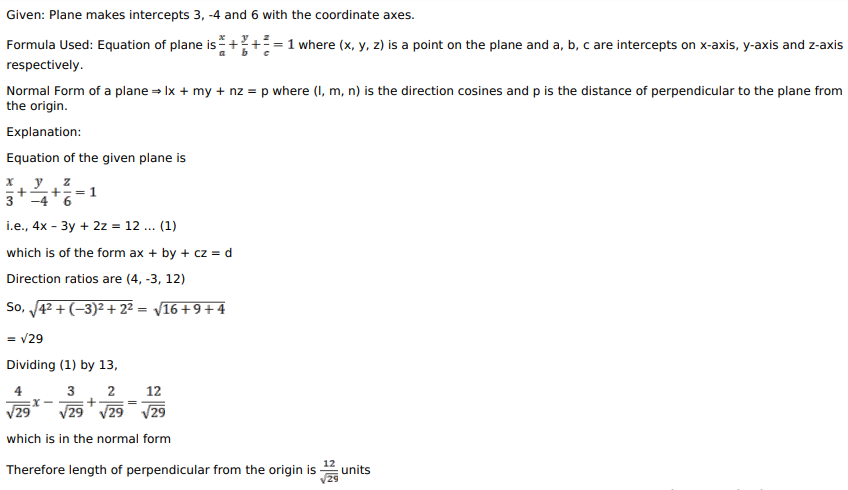# Mark against the correct answer in each of the following:

Question:

Mark against the correct answer in each of the following:

A plane cuts off intercepts $3,-4,6$ on the coordinate axes. The length of perpendicular from the origin to this plane is

A. $\frac{5}{\sqrt{29}}$ units

B. $\frac{8}{\sqrt{29}}$ units

C. $\frac{6}{\sqrt{29}}$ units

D. $\frac{12}{\sqrt{29}}$ units

Solution: